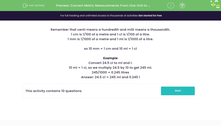# Convert Metric Measurements From One Unit to Another

In this worksheet, students convert metric measurements from one unit to another.Key stage:  KS 2

Curriculum topic:   Measurement

Curriculum subtopic:   Convert Between Metric Measurements

Difficulty level:#### Worksheet Overview

Remember that centi means a hundredth and milli means a thousandth.

1 cm is 1/100 of a metre and 1 cl is 1/100 of a litre.

1 mm is 1/1000 of a metre and 1 ml is 1/1000 of a litre.

so 10 mm = 1 cm and 10 ml = 1 cl

Example

Convert 24.5 cl to ml and l.

10 ml = 1 cl, so we multiply 24.5 by 10 to get 245 ml.

245/1000 = 0.245 litres

Answer: 24.5 cl = 245 ml and 0.245 l

### What is EdPlace?

We're your National Curriculum aligned online education content provider helping each child succeed in English, maths and science from year 1 to GCSE. With an EdPlace account you’ll be able to track and measure progress, helping each child achieve their best. We build confidence and attainment by personalising each child’s learning at a level that suits them.

Get started